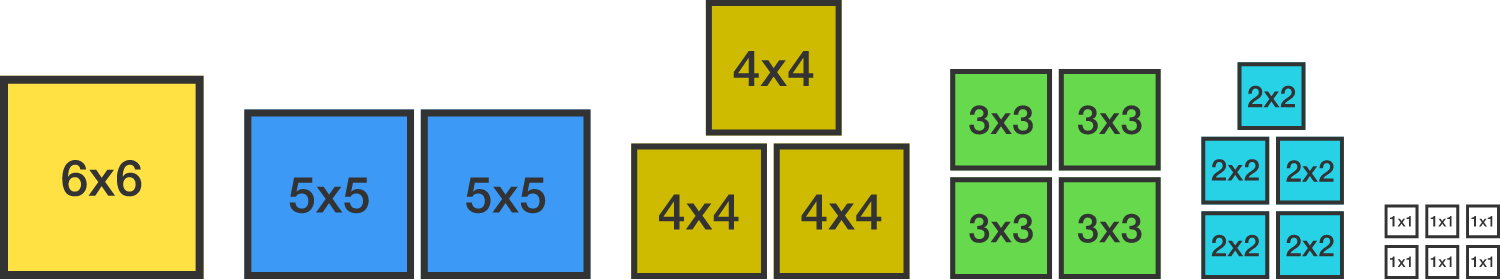# Build a square from squares?

Geometry Level 1Can you use all of the pieces to build a large square? And if possible, what is its side length?

• one $6 \times 6$ square
• two $5 \times 5$ squares
• three $4 \times 4$ squares
• four $3 \times 3$ squares
• five $2 \times 2$ squares
• six $1 \times 1$ squares
×

Problem Loading...

Note Loading...

Set Loading...